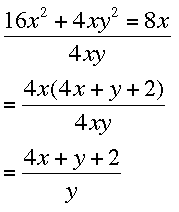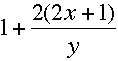SEARCH HOMEMath Central Quandaries & QueriesQuestion from Ricardo, a student: how can i solve that problem of polynomial division? 16x^2+4xy^2+8x divided by 4xyHi Ricardo,

The first thing I notice is that 4x is a common factor in the numerator. ThusI would probab;y leave the ratio in this form but you might write is asPennyMath Central is supported by the University of Regina and The Pacific Institute for the Mathematical Sciences.